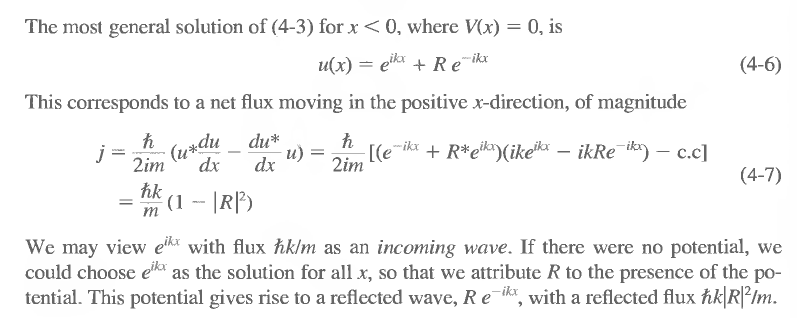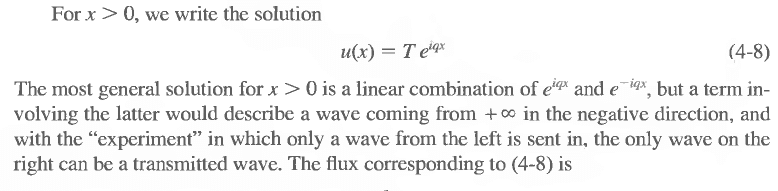# Why does only e^ikx+Re^-ikx solve the TISE for a potential step?

## Homework Statement

Solve the time-independent Schrödinger equation in one dimension for a potential step, i.e. V(x) = 0 for x<0 and V(x) = V_0 for x>0.

## Homework Equations

$$- \frac{\hbar^2}{2m} \frac{d^2u(x)}{dx^2} + V(x)u(x) = Eu(x)$$

## The Attempt at a Solution

Rewrite as (4-3)

$$\frac{d^2u(x)}{dx^2} + \frac{2m}{\hbar^2}( E - V(x) )u(x) = \frac{d^2u(x)}{dx^2} + k^2( 1 - V(x)/E )u(x) = 0$$

Looking at x<0, i.e. V(x)=0:

$$\frac{d^2u(x)}{dx^2} + k^2u(x) = 0 \Leftrightarrow u(x) = Ae^{ikx} + Be^{ikx}$$

The derivation in my textbook claims, at this step:Is it implicit that all multiples of the suggested u(x) are solutions (for x<0)? Furthermore, from the paragraph on the probability flux, it looks like |R(x)| < 1. Why?

It seems like I'm missing some assumption. Why would the x<0 case be different from the case of a free particle?

Edit: I'm having a similar problem with x>0.Gasiorowicz talks about the waves having a direction. What is meant by this? Why is the term e^-iqx dismissed?

Last edited:

## Answers and Replies

im assuming here that you have done a previous course on wave physics?
ok in very simple terms, the solution describes an unbound particle moving in some direction with wavenumber k, where k is some vector in n-dimensions, describing the direction of the motion of the particle. if you look closely at your original equation:
−ℏ22md2u(x)dx2+V(x)u(x)=Eu(x)
u have a second derivative of a function on the left and on the right the same function with coefficient E-V. you know that V is positive or zero, so you will find that for an incoming particle upon the barrier (x=0) should E>V, then the solution will be an imaginary complex exponential. in this case your wavefunction u is not normalisable (find out why!) and hence you must describe the particle by a dirac-delta function.
so basically the only way the wavefunction will make physical sense is to say it is like a bullet moving on some well defined trajectory. hence you can assume the particles are like waves incident on some boundary, with some probability of reflection (R) and some probability of transmission (T) which should complement one another to give 1 (100%).
hope this will help

Last edited:
Thank you ardie, that makes it clearer.

vela
Staff Emeritus
Science Advisor
Homework Helper
Education Advisor
If you apply the momentum operator
$$\hat{p}=\frac{\hbar}{i}\frac{\partial}{\partial x}$$
to the state ψ(x)=eikx, you get
$$\hat{p}\psi(x) = \frac{\hbar}{i}\frac{\partial}{\partial x}e^{ikx} = (\hbar k)\psi(x)$$
This tells you ψ(x) is a momentum eigenstate, where the momentum of the particle is equal to $\hbar k$.

In both regions, the general solution is of the form Aeikx+Be-ikx, which you can, in light of the above, interpret as particles moving in the positive direction and negative direction. Now it's essentially a matter of applying boundary conditions. In this particular problem, you're interested in what happens if a particle is incident on the potential step when coming from the left. You would expect a reflected wave, in the x<0 region, and a transmitted wave, in the x>0 region. Consequently, you keep both terms for x<0, one representing the incident wave and one representing the reflected wave. For x>0, you only have the transmitted wave, so you drop the e-ikx term.

Much clearer! Looking at the momentum makes it more concrete. Thank you very much.